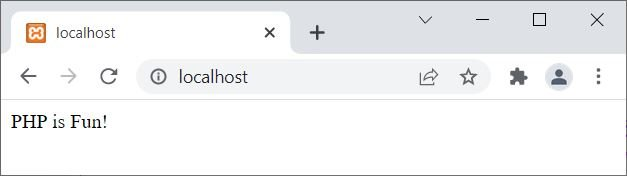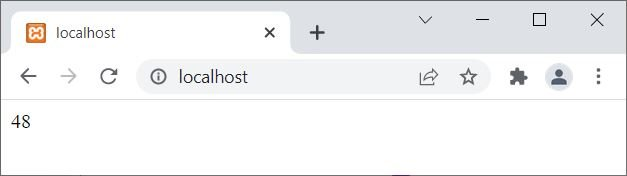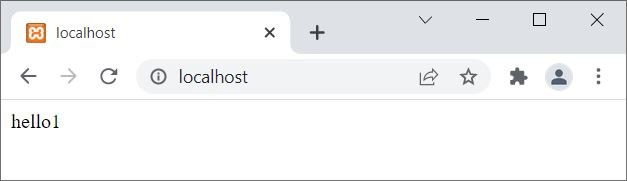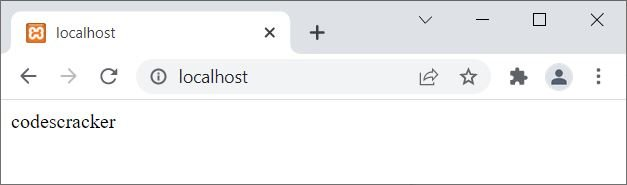# PHP print statement

The PHP print statement is used to output some data on the screen or on the web. For example:

```<?php
print "PHP is Fun!";
?>```

The output of the above PHP example is:The same (previous) example can also be created in this way:

```<?php
\$x = "PHP is Fun!";
print \$x;
?>```

Let me create the same example another way:

```<?php
\$x = "PHP is Fun!";
print(\$x);
?>```

It produces exactly the same output as the previous example because (\$x) is evaluated as "PHP is Fun!" (which is a valid expression).

Note: But let me tell you one thing: use parentheses only when you need to give priority to an expression being executed first. For example:

```<?php
print(1+3) * 12;
?>```

The output of the above PHP example is:In the above example, if you remove the parentheses, then the output should be 37. This is the situation where we need to use parentheses. Otherwise, print does not requires any parentheses to output the data on screen.

## PHP print statement example

```<?php
\$x = 24;
print \$x;
print "<hr>";

\$x = 18.93;
print \$x;
print "<hr>";

\$x = "Hey!";
print \$x;
print "<hr>";

\$x = "Hey,<br>is everything OK?";
print \$x;
?>```

The output of the above PHP example is:Note: Use echo instead of print to output data on the screen. But since the print statement always returns 1, use print when needed to execute an expression. For example:

```<?php
\$x = '';
print print \$x;
?>```

This example will produce 1. Let me create another example based on this scenario:

```<?php
\$x = 'hello';
print print \$x;
?>```

The output of the above PHP example is:Now the time is to create one last example on a print statement or keyword to complete the tutorial on it:

```<?php
if(print "codes")
{
echo "cracker";
}
?>```

The output of the above PHP example is:### Advantages of print statement in PHP

• Simple to Use: There are no special coding skills needed to use the "print" statement. It is a crucial component of PHP and is widely used in web development.
• Quick Output: To output strings or variables to the browser, the "print" statement is frequently used. It is quick and effective, just like the "echo" statement.
• Compatible with HTML: The "print" statement can be used to output HTML code directly to the browser because it is also HTML-compatible. This facilitates the development of dynamic web pages that can be instantly updated.

### Disadvantages of print statement in PHP

• "print" statement are less expansive than those for the "echo" statement. Both string concatenation and the use of multiple parameters are not supported.
• Combining PHP and HTML: Similar to the "echo" statement, it can be challenging to determine where the PHP code ends and the HTML code begins when using the "print" statement to output HTML code. If errors do occur, this may make it more difficult to debug the code.
• Security Risks: If user input is not properly sanitized before being output to the browser, the "print" statement may also be subject to security risks. This may make it possible for malicious code to be injected onto the page, creating security holes.

PHP Online Test

« Previous Tutorial Next Tutorial »